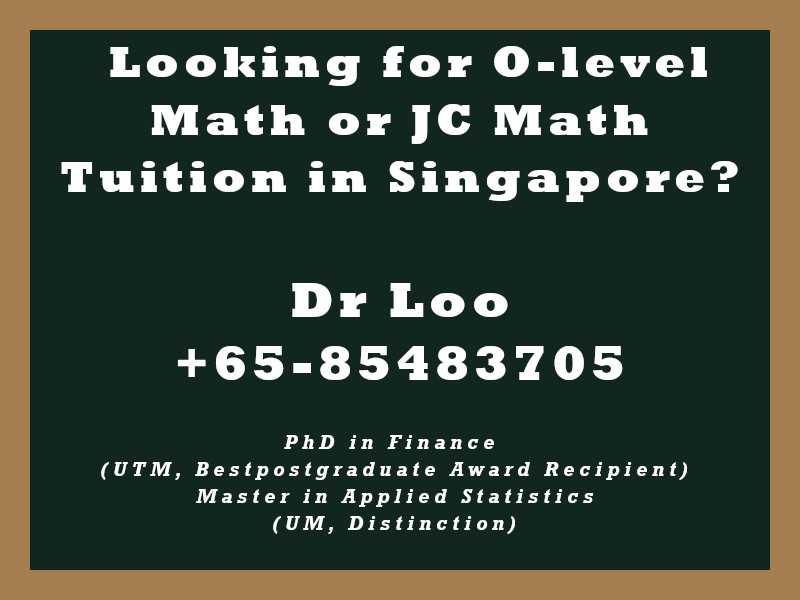+65-85483705

# Sequences and Series

Question

A. Solve the following questions related to a series.

1. If the sum of the nth terms in an arithmetic progression is Sn = 3n + n2, find the 5th term of the series.
2. In an arithmetric progression, the 10th term is 21 while the 12nd term is 25. Find the common difference (d) and also the first two terms of the series.

Guide:

1. The formula to find the sum of the nth terms in an arithmetic progression is Sn = n/2(2a + (n-1)d)
2. The formula to find the nth term in an arithmetic progression is: an = a + (n-1)d
3. When taking H2 mathematics class, you will learn about things like concept of sequence and series for finite cases and infinite cases, sum and difference of two series, use of sigma notation and other interesting features of sequence and series.

1. d = 2, a1 = 3, a2 = 5
2. a5 = 12

## About the Singapore Math Tutor - Dr Loo

I am a PhD holder with 9 years of teaching experience on teaching quantitative subjects at secondary school and university levels. My expertise are mathematics, statistics, econometrics, finance and machine learning.

Currently I do provide consultation and tuition for math tuition in Singapore. If you are looking for secondary math tutor in Singapore, JC H2 math tutor or statistics tutor in Singapore, please feel free to contact me at +65-85483705 (SMS/Whatsapp).

Among the Singapore math tuition that I provide, this includes Singapore secondary math tuition (E-math tuition & A-math tuition) and also JC Math Tuition (H1 Math Tuition & H2 Math Tuition). On the other hand, my statistics tuition in Singapore mostly focus on pre-university level until postgraduate level. For those who are looking for online tutoring service, the online O-level math tuition and online A-level math tuition are also available.